# 算法-动态规划 Dynamic Programming--从菜鸟到老鸟算法 同时被 2 个专栏收录53 篇文章 7 订阅

## 动态规划算法的核心A * "1+1+1+1+1+1+1+1 =？" *

A : "上面等式的值是多少"
B : *计算* "8!"

A *在上面等式的左边写上 "1+" *
A : "此时等式的值为多少"
B : *quickly* "9!"
A : "你怎么这么快就知道答案了"
A : "只要在8的基础上加1就行了"
A : "所以你不用重新计算因为你记住了第一个等式的值为8!动态规划算法也可以说是 '记住求过的解来节省时间'"


# 动态规划算法的两种形式

Fibonacci (n) = 1;   n = 0

Fibonacci (n) = 1;   n = 1

Fibonacci (n) = Fibonacci(n-1) + Fibonacci(n-2)


public int fib(int n)
{
if(n<=0)
return 0;
if(n==1)
return 1;
return fib( n-1)+fib(n-2);
}
//输入6
//输出：8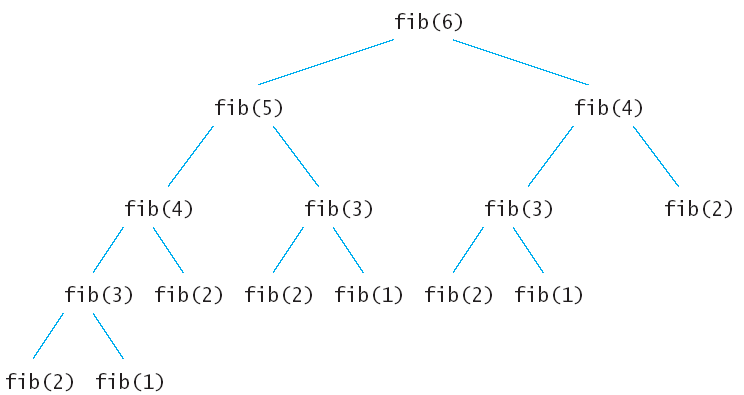## ①自顶向下的备忘录法

public static int Fibonacci(int n)
{
if(n<=0)
return n;
int []Memo=new int[n+1];
for(int i=0;i<=n;i++)
Memo[i]=-1;
return fib(n, Memo);
}
public static int fib(int n,int []Memo)
{

if(Memo[n]!=-1)
return Memo[n];
//如果已经求出了fib（n）的值直接返回，否则将求出的值保存在Memo备忘录中。
if(n<=2)
Memo[n]=1;

else Memo[n]=fib( n-1,Memo)+fib(n-2,Memo);

return Memo[n];
}


## ②自底向上的动态规划

public static int fib(int n)
{
if(n<=0)
return n;
int []Memo=new int[n+1];
Memo=0;
Memo=1;
for(int i=2;i<=n;i++)
{
Memo[i]=Memo[i-1]+Memo[i-2];
}
return Memo[n];
}


public static int fib(int n)
{
if(n<=1)
return n;

int Memo_i_2=0;
int Memo_i_1=1;
int Memo_i=1;
for(int i=2;i<=n;i++)
{
Memo_i=Memo_i_2+Memo_i_1;
Memo_i_2=Memo_i_1;
Memo_i_1=Memo_i;
}
return Memo_i;
}


## 动态规划小试牛刀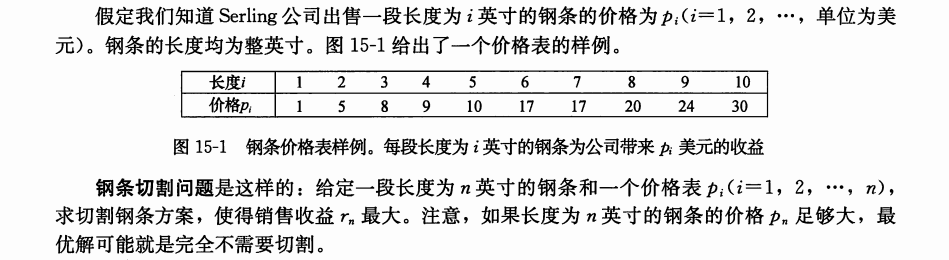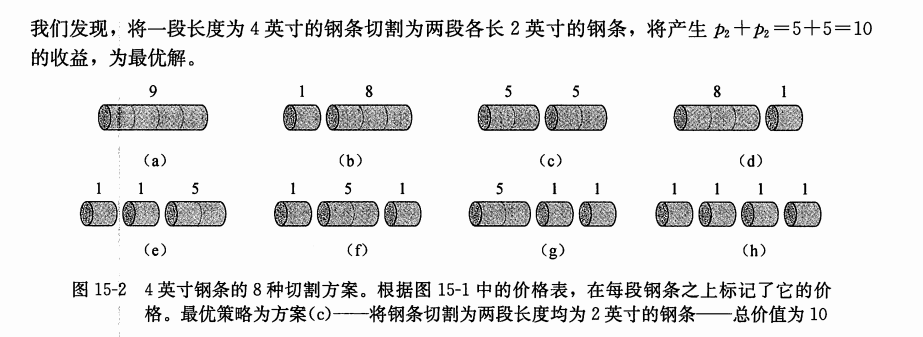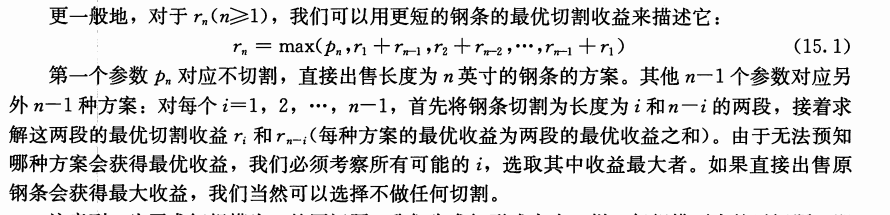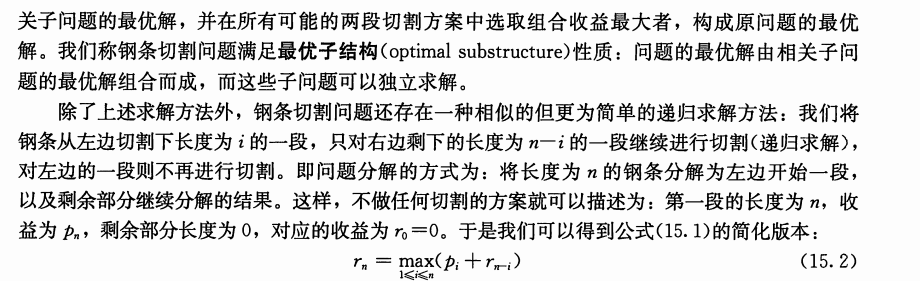①递归版本

public static int cut(int []p,int n)
{
if(n==0)
return 0;
int q=Integer.MIN_VALUE;
for(int i=1;i<=n;i++)
{
q=Math.max(q, p[i-1]+cut(p, n-i));
}
return q;
}


②备忘录版本

public static int cutMemo(int []p)
{
int []r=new int[p.length+1];
for(int i=0;i<=p.length;i++)
r[i]=-1;
return cut(p, p.length, r);
}
public static int cut(int []p,int n,int []r)
{
int q=-1;
if(r[n]>=0)
return r[n];
if(n==0)
q=0;
else {
for(int i=1;i<=n;i++)
q=Math.max(q, cut(p, n-i,r)+p[i-1]);
}
r[n]=q;

return q;
}


③自底向上的动态规划

public static int buttom_up_cut(int []p)
{
int []r=new int[p.length+1];
for(int i=1;i<=p.length;i++)
{
int q=-1;
//①
for(int j=1;j<=i;j++)
q=Math.max(q, p[j-1]+r[i-j]);
r[i]=q;
}
return r[p.length];
}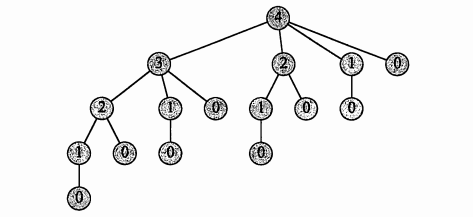①最优子结构

②重叠子问题

# 动态规划的经典模型

## 线性模型

**【例题1】**在一个夜黑风高的晚上，有n（n <= 50）个小朋友在桥的这边，现在他们需要过桥，但是由于桥很窄，每次只允许不大于两人通过，他们只有一个手电筒，所以每次过桥的两个人需要把手电筒带回来，i号小朋友过桥的时间为T[i]，两个人过桥的总时间为二者中时间长者。问所有小朋友过桥的总时间最短是多少。T = minPTime * (N-2) + (totalSum-minPTime)

a }

## 区间模型

【例题2】给定一个长度为n（n <= 1000）的字符串A，求插入最少多少个字符使得它变成一个回文串。

1、在A[j]后面添加一个字符A[i]；

2、在A[i]前面添加一个字符A[j]；

d[i][j] = min{ d[i+1][j], d[i][j-1] } + 1; (每次状态转移，区间长度增加1)

## 背包模型

**【例题3】**有N种物品（每种物品1件）和一个容量为V的背包。放入第 i 种物品耗费的空间是Ci，得到的价值是Wi。求解将哪些物品装入背包可使价值总和最大。f[i][v]表示前i种物品恰好放入一个容量为v的背包可以获得的最大价值。决策为第i个物品在前i-1个物品放置完毕后，是选择放还是不放，状态转移方程为：

f[i][v] = max{ f[i-1][v], f[i-1][v – Ci] +Wi }

## 动态规划题集整理

1、最长单调子序列
Stock Exchange ★★☆☆☆

2、最大M子段和
Max Sum ★☆☆☆☆

3、线性模型
Skiing ★☆☆☆☆

# 弄懂动态规划问题的基本原理和动态规划问题的几个常见的模型，对于解决大部分的问题已经足够了。希望能对大家有所帮助，转载请标明出处https://blog.csdn.net/u013309870/article/details/75193592，创作实在不容易，这篇博客花了我将近一个星期的时间。参考文献

1.算法导论

08-1127万+08-255万+
06-1616万+
11-134万+
07-25452
10-1123万+
08-181万+
05-051万+
06-031万+
09-1521
11-132万+
08-071万+
04-181304
02-2749
07-241万+
©️2021 CSDN 皮肤主题: Age of Ai 设计师:meimeiellie点击重新获取扫码支付1.余额是钱包充值的虚拟货币，按照1:1的比例进行支付金额的抵扣。
2.余额无法直接购买下载，可以购买VIP、C币套餐、付费专栏及课程。余额充值